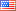Question-and-Answer Resource for the Building Energy Modeling Community
Get s tarted with the Help page

# Revision history [back]

### doe21e .bin output

Hi I need to export the variable to a file in doe21e

PLOTER2 = REPORT-BLOCK
VARIABLE-TYPE = GLOBAL
VARIABLE-LIST = (4) .. $DRY BULB TEMPERATURE PLOTD = HOURLY-REPORT REPORT-SCHEDULE=PLTSCH REPORT-BLOCK = (PLOTER2) OPTION = PRINT$HOURLY-DATA-SAVE= FORMATTED
AXIS-ASSIGN = (1)
AXIS-TITLES = (*DRY BULB TEMPERATURE*)
AXIS-MAX = (120)
AXIS-MIN = (0,0)
DIVIDE = (1) ..


however, u cant find where the bin file when i choose **OPTION = BINARY-FILE" where is .bin file and how to parse it with excel ? thanks

### doe21e .bin output

Hi I need to export the variable to a file in doe21e

PLOTER2 = REPORT-BLOCK
VARIABLE-TYPE = GLOBAL
VARIABLE-LIST = (4) .. $DRY BULB TEMPERATURE PLOTD = HOURLY-REPORT REPORT-SCHEDULE=PLTSCH REPORT-BLOCK = (PLOTER2) OPTION = PRINT$HOURLY-DATA-SAVE= FORMATTED
AXIS-ASSIGN = (1)
AXIS-TITLES = (*DRY BULB TEMPERATURE*)
AXIS-MAX = (120)
AXIS-MIN = (0,0)
DIVIDE = (1) ..


however, u i cant find where the bin file when i choose **OPTION = BINARY-FILE" where is .bin file and how to parse it with excel ? thanks3 retagged__AmirRoth__4388 ●5 ●16 http://bleedinggreenna...

### doe21e .bin output

Hi I need to export the variable to a file in doe21e

PLOTER2 = REPORT-BLOCK
VARIABLE-TYPE = GLOBAL
VARIABLE-LIST = (4) .. $DRY BULB TEMPERATURE PLOTD = HOURLY-REPORT REPORT-SCHEDULE=PLTSCH REPORT-BLOCK = (PLOTER2) OPTION = PRINT$HOURLY-DATA-SAVE= FORMATTED
AXIS-ASSIGN = (1)
AXIS-TITLES = (*DRY BULB TEMPERATURE*)
AXIS-MAX = (120)
AXIS-MIN = (0,0)
DIVIDE = (1) ..


however, i cant find where the bin file when i choose **OPTION = BINARY-FILE" where is .bin file and how to parse it with excel ? thanks

### doe21e .bin output

Hi I need to export the variable to a file in doe21e

PLOTER2 = REPORT-BLOCK
VARIABLE-TYPE = GLOBAL
VARIABLE-LIST = (4) .. $DRY BULB TEMPERATURE PLOTD = HOURLY-REPORT REPORT-SCHEDULE=PLTSCH REPORT-BLOCK = (PLOTER2) OPTION = PRINT PRINT$HOURLY-DATA-SAVE= FORMATTED
AXIS-ASSIGN = (1)
AXIS-TITLES = (*DRY BULB TEMPERATURE*)
AXIS-MAX = (120)
AXIS-MIN = (0,0)
DIVIDE = (1) ..


however, Updated: I have gotten the CEC1_01.DAT file but it adds more lines each run so i cant find where need to remove .DAT each run. Have any way to update not append the bin file when i choose **OPTION = BINARY-FILE" where is .bin file and how to parse it with excel ? .DAT file thanks